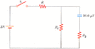# RC circuit problem

• SamTsui86

## Homework Statement

In Figure P18.50, suppose that the switch has been closed for a length of time sufficiently long enough for the capacitor to become fully charged. (R = 12.0 k, R2 = 17.0 k, R3 = 1.00 k, V = 9.70 V)

The picture is under attachment

a) Find the steady-state current in each resistor.
I1 = µA?
I2 = µA?
I3 = µA ?

(b) Find the charge on the capacitor.
µC ?

R = V / I

q = Q e^(-t/RC)

## The Attempt at a Solution

Well, since after the switch is closed, I figured all 9.7 V goes to the first resistor, so I used R = V / I to get the current for the first one. But it's wrong.

#### Attachments

•p18-50alt.gif
4.9 KB · Views: 453